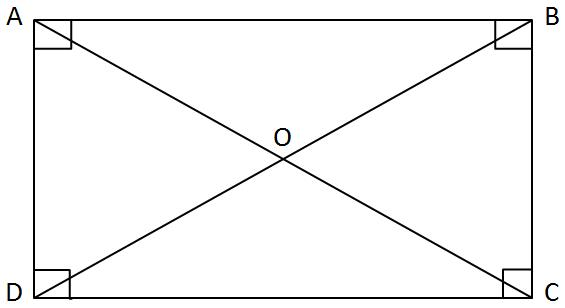Rectangle

Definition: A parallelogram where all the four angles are right angles is called a rectangle.

Properties:1) Opposite sides are equal and parallel to each other.$AB \parallel DC \ and \ AB=DC$$AD \parallel BC \ and \ AD=BC$

2) Each angle measures$90^{\circ} \ i.e.\ \angle A=\angle B=\angle C=\angle D=90^{\circ}$

3) The diagonals are equal i.e.$AC = BC$

4) The diagonals$(AC \ and\ BD)$ bisect each other$AO = OC$$DO = OB$

Theorem: The diagonals of a rectangle are equal.

Given:$ABCD$ is a rectangle and the diagonals are$AC \ and\ BD$

To prove:$AC = BD$

Consider$\Delta BCD \ and\ \Delta ACD$$AD = BC$ (given that it is a rectangle)$DC$ is common$\angle ADC =\angle BCD$ (right angles)

Hence$\Delta ACD \cong \Delta BCD$ (S.A.S axiom)

Therefore$AC = BD$. Hence Proved

Square

A parallelogram which has all sides equal and all four angles are right angle is called a square.Or we can also say that a square is a rectangle that has all four sides equal.

Properties of square:

1) All sides are equal i.e.$AB = BC = CD = DA$

2) Each angle measures$90^{\circ} i.e. \angle A =\angle B =\angle C =\angle D = 90^{\circ}$

3) The diagonals are equal i.e.$AC = BC$

4) The diagonals$(AC \ and\ BD)$ bisect each other$AO = OC\ \& \ DO = OB$

The diagonals intersect at right angles i.e.$AC \perp BD$

Theorem: The diagonals of a square are equal and perpendicular to each other.

To prove:$AC = BD \ and\ AC \perp BD$

Given: Square$ABCD$, Diagonals$AC \ and\ BD \ intersect \ at \ O$

Proof:

Consider$\Delta BCD \ and\ \Delta ACD$$AD = BC$ (given that it is a square)$DC$ is common$\angle ADC = \angle BCD \$ (right angles)

Hence$\Delta ACD \cong \Delta BCD$ (S.A.S axiom)

Therefore$AC = BD$. Hence Proved

Consider$\Delta COD \ and\ \Delta AOD$$OC = OA$ (diagonals of a parallelogram bisect each other)$DO$ is common$D=DC$ (Sides of a triangle)

Hence$\Delta COD \cong \Delta AOD$ (S.S.S axiom)

Hence$\angle DOC=\angle DOA$

We know that$\angle DOC+\angle DOA=180^{\circ}$

Therefore$\angle DOC=\angle DOA=90^{\circ}$

Hence$DO\perp AC \ i.e.\ AC\perp BD$